• 计算残差平方和均值。 以下是残差平方和公式。 package sampling.method;import java.util.HashMap; import java.util.Map; import org.apache.commons.math3.linear.Array2DRowRealMatrix; import org.
以下，是我在写一个算法时中的一小部分，程序给了一个简单的算例。
计算残差平方和的均值。  以下是残差平方和公式。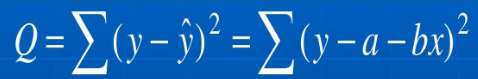package sampling.method;

import java.util.HashMap;
import java.util.Map;
import org.apache.commons.math3.linear.Array2DRowRealMatrix;
import org.apache.commons.math3.linear.RealMatrix;
public class Test {

public static void main(String[] args) {
Map<Integer,RealMatrix > ymatrix=new HashMap<Integer,RealMatrix >();
double[][] y1={{1,2,3,4}};
RealMatrix y1matrix = new Array2DRowRealMatrix(y1);
double[][] y2={{2,3,3,5,7}};
RealMatrix y2matrix = new Array2DRowRealMatrix(y2);
//这里的1和2分别表示类别
ymatrix.put(1, y1matrix);
ymatrix.put(2, y2matrix);
//
Map<Integer,RealMatrix > xMatrixList=new  HashMap<Integer,RealMatrix >();
double[][] x1={{1,2},{3,4},{2,4},{2,4}};
double[][] x2={{1,2},{3,4},{0,0},{5,4},{2,6}};
RealMatrix x1mat = new Array2DRowRealMatrix(x1);
RealMatrix x2mat = new Array2DRowRealMatrix(x2);
xMatrixList.put(1, x1mat);
xMatrixList.put(2, x2mat);
//
Map<Integer,RealMatrix > wMatrixList=new  HashMap<Integer,RealMatrix >();
double[][] w1={{1,2}};
double[][] w2={{2,3}};
RealMatrix w1mat = new Array2DRowRealMatrix(w1);
RealMatrix w2mat = new Array2DRowRealMatrix(w2);
wMatrixList.put(1, w1mat);
wMatrixList.put(2, w2mat);
double sum=0.0;
int dimension=0;
for( int itemnumber : ymatrix.keySet() ){
RealMatrix yscore=ymatrix.get(itemnumber) ;
dimension+=yscore.getColumnDimension();
RealMatrix wxscore=wMatrixList.get(itemnumber).multiply(xMatrixList.get(itemnumber).transpose()) ;
System.out.println(ysbuwxscore.multiply(ysbuwxscore.transpose()));
sum+=sumarray(ysbuwxscore.multiply(ysbuwxscore.transpose()));

}
System.out.println("残差平方和均值为："+sum/dimension);
}
private static double sumarray(RealMatrix a){
double[][] arr=a.getData();
double sum = 0.0;
for (int i = 0; i < arr.length; i++) {
for (int j = 0; j < arr[i].length; j++) {
sum += arr[i][j];
}
}
return sum;
}
}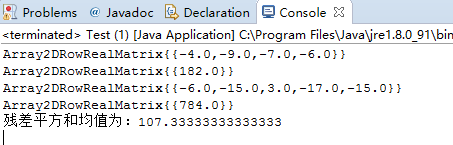展开全文java 算法
• 针对美籍罗马尼亚著名数论专家Florentinsamarandache在onlypro-lomnotsolution,中提出的数字之和函数的性质问题,研究了非零数字倒数平方和函数均值问题。通过猜想、数学归纳和推理论证等方法,得出在一个正整数的n...
• 通常的说法是：“方差等于均方值减去均值平方”Var = E[(X-μ)²] = E[X²-2Xμ+μ²] = E(X²)-2μ²+μ² = E(X²)-μ² (*)(*) 式的含义就是：“方差等于均方值减去均值平方
通常的说法是：“方差等于均方值减去均值的平方”Var = E[(X-μ)²] = E[X²-2Xμ+μ²] = E(X²)-2μ²+μ² = E(X²)-μ²    (*)(*) 式的含义就是：“方差等于均方值减去均值的平方”
展开全文• 给出了有关n进制数位数码之平方和的函数均值的一个精确的计算公式。
• 巧得cos平方均值——妙啊！ cos平方的平均值应该等于sin平方的平均值， 而这两个相加=1， 因此分别为1/2。
巧得cos平方的均值——妙啊！
cos平方的平均值应该等于sin平方的平均值，
而这两个相加=1，
因此分别为1/2。
展开全文• 设ψ（n）是Dedekind函数。以E（χ）表示式 的渐近公式中的误差项，本文研究了E（χ的加权平方积分均值
• 课程作业练习 K均值聚类（最小误差平方和准则） 这是学习机器学习课程的一个作业，自己的学习记录，利用python实现，依据图中的算法思路完成，初学，还需要很多改进。 首先生成了5类正态分布的点，对这些点进行聚类...
课程作业练习 K均值聚类（最小误差平方和准则）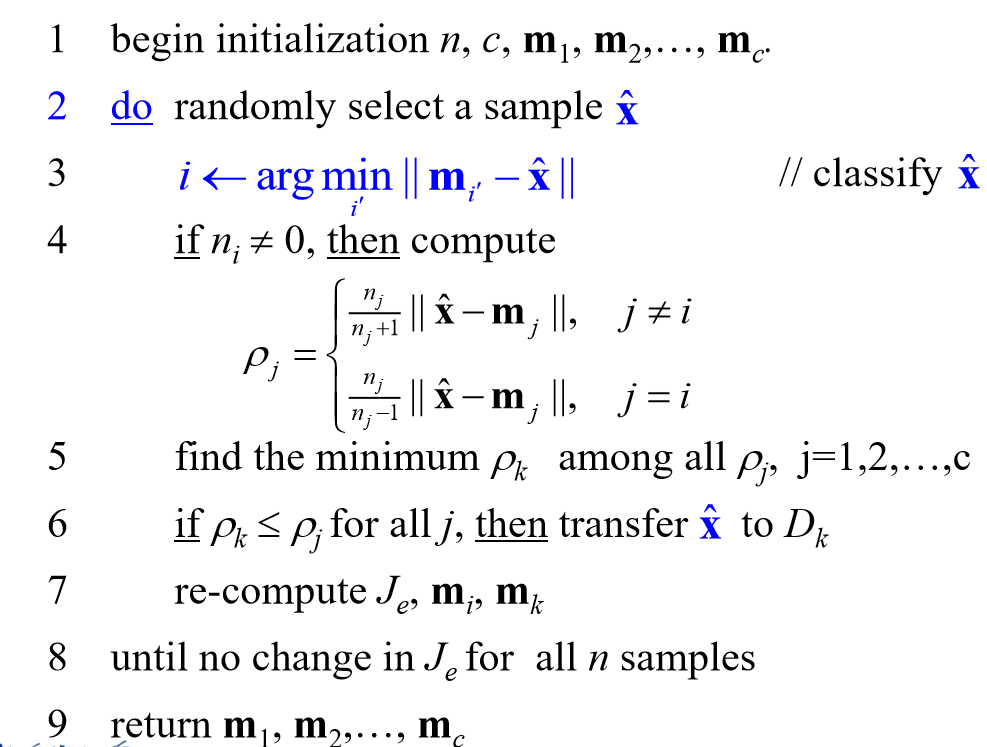这是学习机器学习课程的一个作业，自己的学习记录，利用python实现，依据图中的算法思路完成，初学，还需要很多改进。 首先生成了5类正态分布的点，对这些点进行聚类。 其中的NMI函数的实现来源于网络，侵权即删。 代码可用，循环5次取均值


#by gao
import numpy as np
import random
import collections
import matplotlib.pyplot as plt
import math

np.random.seed(11)
sigma=[[1, 0], [0, 1]]
mu1=(1,-1)
x1=np.random.multivariate_normal(mu1,sigma,200)
mu2=(5,-4)
x2=np.random.multivariate_normal(mu2,sigma,200)
mu3=(1,4)
x3=np.random.multivariate_normal(mu3,sigma,200)
mu4=(6,4)
x4=np.random.multivariate_normal(mu4,sigma,200)
mu5=(7,0.0)
x5=np.random.multivariate_normal(mu5,sigma,200)
lists=(x1,x2,x3,x4,x5)
x_all=[]
X_all=[]
for i in lists:
x_all.extend(i)
X_all.append(i)

def Euc_distance(x1,x2):
l1=((abs(np.linalg.norm(x1)-np.linalg.norm(x2)))**2)**0.5
return l1
def initial_point(k,x):
point_list=[random.choice(x) for choices in range(k)]
return point_list
def sel_point(k,x,point_list):
all_f=[]
for i1 in x:#循环每一个点
f=[]
for i in point_list:#循环簇心中的每一个点
f.append(Euc_distance(i1,i))#存放集合中一个点到几个簇心的距离
b = f.index(min(f))
all_f.append([b,i1])
print(point_list)
the_one=[]
ke=0
for ke in range(k):
the_two=[]
for KK in all_f:
if KK==ke:
the_two.append(KK)
the_one.append(the_two)
each=[len(gg) for gg in the_one]
print('划分数据集合',each)
return the_one
def showing(the_one,point_list):
all_temps=the_one
color=('ro','ko','go','co','bo','yo','mo')
oo=0
for i in range(len(all_temps)):
oo+=1
for t in range(len(all_temps[i])):
plt.plot(all_temps[i][t],all_temps[i][t],color[oo],marker='*',alpha=0.8)
oo=0
for i4 in point_list:
oo+=1
plt.plot(i4, i4,color='0.5',marker='o',markersize=10)
plt.show()
def pj(n,mydata,center,means,k,the_one):#n:该类样本的个数 center:该类的类中心 means:这些样本的均值 k:聚类个数 the_one:聚类集合
dic=dict()
for xj in mydata:
pj=[]
for g in range(len(the_one)):
if xj  in np.array(the_one[g]):
pj1=(np.linalg.norm(xj-center[g]))*n[g]/(n[g]+1)
pj.append(pj1)
else:
pj1=(np.linalg.norm(xj-center[g]))*n[g]/(n[g]-1)
pj.append(pj1)
inx=pj.index(min(pj))
if inx in dic.keys():
dic[inx].append(xj)
else:
dic[inx]=[]
dic[inx].append(xj)
lits=[]
for gdf in dic.keys():
lits.append(dic[gdf])
return lits,dic#返回聚类各集合
def cacu_center(lit):
c_heart=[]
wcf_list=[]
numbers=[]
for ie in range(len(lit)):
sumed,ty_means,wcf_sum=0,0,0
numbers.append(len(lit[ie]))
for ie2 in range(len(lit[ie])):
sumed=sumed+np.array(lit[ie][ie2])
ty_means=np.linalg.norm(sumed/(len(lit[ie])))#重新计算各簇均值
c_heart.append(sumed/(len(lit[ie])))#重新计算簇心
wcf_list.append(round(ty_means,4))
return numbers,c_heart,wcf_list
def K_means(k,x,times):
ini=initial_point(k,x)
setlist=sel_point(k,x,ini)
showing(setlist,ini)
print('初始聚类点')
numbers,c_heart,means=cacu_center(setlist)
next_numbers,next_c_heart,next_meas=cacu_center(lit)
print('第1次迭代')
nn=1
while np.linalg.norm(c_heart) != np.linalg.norm(next_c_heart) and nn<times:
nn+=1
numbers,c_heart,means=next_numbers,next_c_heart,next_meas
next_numbers,next_c_heart,next_meas=cacu_center(lit)
print('第',nn,'次迭代')
print('迭代完成')
showing(lit,next_c_heart)
def NMI_c(A,B):
#样本点数
total = len(A)
A_ids = set(A)
B_ids = set(B)
#互信息计算
MI = 0
eps = 1.4e-45
for idA in A_ids:
for idB in B_ids:
idAOccur = np.where(A==idA)
idBOccur = np.where(B==idB)
idABOccur = np.intersect1d(idAOccur,idBOccur)
px = 1.0*len(idAOccur)/total
py = 1.0*len(idBOccur)/total
pxy = 1.0*len(idABOccur)/total
MI = MI + pxy*math.log(pxy/(px*py)+eps,2)
# 标准化互信息
Hx = 0
for idA in A_ids:
idAOccurCount = 1.0*len(np.where(A==idA))
Hx = Hx - (idAOccurCount/total)*math.log(idAOccurCount/total+eps,2)
Hy = 0
for idB in B_ids:
idBOccurCount = 1.0*len(np.where(B==idB))
Hy = Hy - (idBOccurCount/total)*math.log(idBOccurCount/total+eps,2)
MIhat = 2.0*MI/(Hx+Hy)
return MIhat
from sklearn import metrics
import math
ff=[]
x_lable=[]
y_lable=[]
for g in range(len(X_all)):
f=[]
gf=[]
for gg in X_all[g]:
f.append(xg)
gf.append([g,xg])
fs=collections.Counter(f)
gr=[]
gc=[]
for v in fs.keys():
gc.append(v)
gr.append(fs[v])
ff.append(max(gr))
ffindex=gr.index(max(gr))
ffindex=gc[ffindex]
cv=[g if i ==ffindex else i for i in np.array(gf).T]
x_lable.extend(np.array(gf).T)
y_lable.extend(np.array(cv))
acc=np.array(ff).sum() / len(x_all)
NMI=NMI_c(x_lable,y_lable)
return acc,NMI
if __name__ == '__main__':
me=[]
me2=[]
for i in range(5):
me.append(Acc)
me2.append(NMI)
print(np.array(me).mean(),np.array(me2).mean())


运行结果如下： 会先生成初始选点的类图，最后输出聚类后的图、Acc和NMI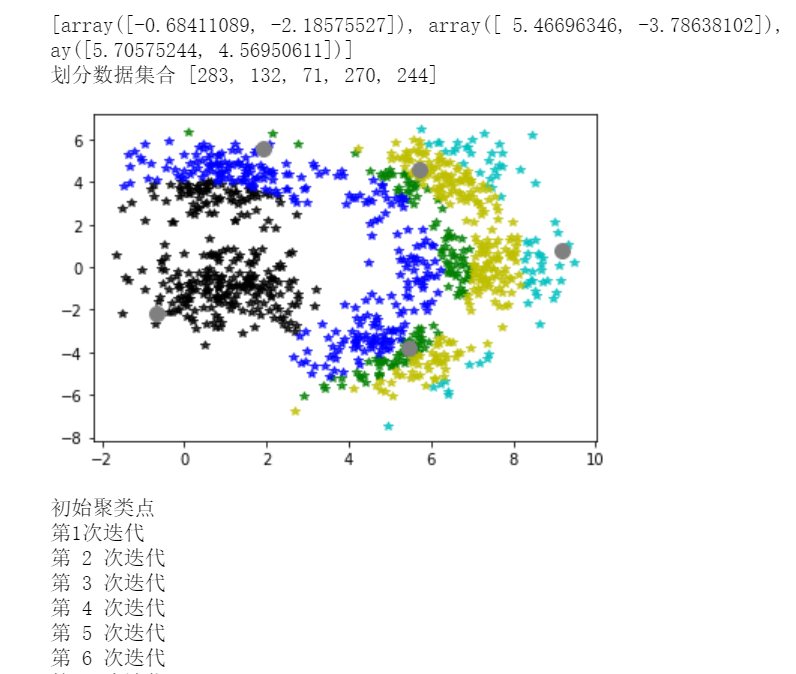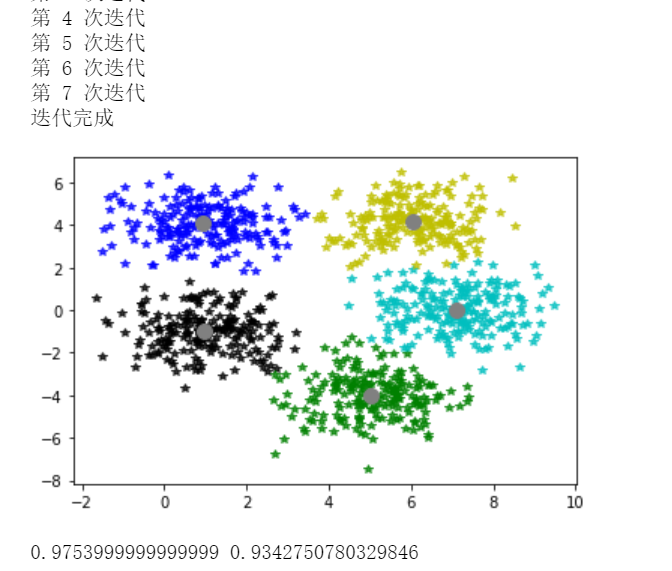展开全文聚类 python 聚类算法 机器学习 算法
• C均值算法又叫K-Means算法，其基本思想是，通过迭代找到k个聚类的一种划分方案，使得用这k个聚类的均值来表示相应各类样本时所得到的总体误差最小，所以K-Means是一种基于最小误差平方和准则的聚类算法。由于它在...
• 充分考虑随机量测误差的正态分布特点，提出了基于...分析了文献错误参数综合残差平方和均值辨识法存在的问题，论证了所提方法在消除量测误差影响和确定均值样本数两方面的优势，并通过仿真分析验证了所提方法的有效性。
• 整理了四种常用的聚类方法，自用，现供大家参考 K均值聚类方法中 聚类个数是用了factoextra包中的fviz_nbclust函数 并用此包中的画图函数绘制了拐点图，结果较为直观...系统聚类包括最大距离法、类平均法和离差平方和
• 回归平方和 ESS，残差平方和 RSS，总体平方和 TSS 总变差 （TSS）：被解释变量Y的观测值与其平均值的离差平方和（总平方和）(说明 Y 的总变动程度） 解释了的变差 （ESS）：被解释变量Y的估计...
• 在一般的数学统计过程中，为了求得方差，需要预先知道所有的数据项，然后通过求均值，再通过遍历所有数据项计算平方和的方式求得方差。 但是在大数据、流式处理的场景，是无法预先知道所有数据项的，经常需要在任意...方差递推 推导
• 参考： Welford, BP，“关于计算校正平方和和乘积的方法的说明。” 技术计量学，4(3)，第 419-420 页，1962 年。 注意：在当前实现中，移动历史被保持（类似于移位寄存器）。 这可能仍然代表相当大的计算负担。 ...matlab
• %% 均值计算，如果X是一个矩阵，则其均值是一个向量组。mean(X,1)为列向量的均值，mean(X,2)为行向量的均值。 %若要求整个矩阵的均值，则为mean(mean(X))。也可使用mean2函数：mean2(X) mean(X,1)%我需要的列均值 ...
• c-均值聚类又称硬C-均值聚类，该算法把N个向量聚为C个类，聚类准则是使每一聚类中，样本点到该类别的中心的距离的平方和最小。算法及代码，适合初学者使用。
• 方差：代表一种平均功率的偏差，即将所有点平均值偏差的平方相加再做平均： 需要注意的是，如公式所示，偏差功率后求平均时除以N-1而不是N。这是由于均值μ是估计值，当N越小时均值u的偏差越大。计算均值的...
• 　模糊的c均值聚类算法：-------- 一种模糊聚类算法，是k均值聚类算法的推广形式，隶属度取值为[0 1]区间内的任何一个数，提出的基本根据是“类内加权误差平方和最小化”准则； 这两个方法都是迭代求取最终的聚类...
• 1、均值 数学定义： Matlab函数：mean 如果X是一个矩阵，则其均值是一个向量组。mean(X,1)为列向量的均值，mean(X...按求取方差公式的不同，方差分为两种：biased（有效估计的方差）unbiased（无偏估计的方差...标准差
• ## K均值聚类的理解和实现

万次阅读 多人点赞 2018-10-27 11:23:08
目录 1. 距离的测度 ...2.1 K均值的原理实现 2.2 K均值的缺点 2.3 K均值改进 3. 算法实现 3.1 获取样本 3.2 协方差逆阵方根的计算方法 3.3 聚类实验 3.3.1 一般的K均值聚类 3.3.2 基于马氏距离...opencvC语言
• 对图的度平方和的下界进行...利用均值不等式及图中度序列的关系，给出了图G的度平方和的两个下界，并确定了达到这两个下界的极图。同时也给出了度平方和下界的简单应用，用它们来确定一个图及其补图中三角形的总个数。
• 图像处理中均值和方差计算优化 一、均值和方差的普通优化 图像处理中，有时候会需要计算图像某区域的均值和方差。在我之前的博客中《图像比较之模板匹配》，对计算方差有做简化计算的介绍。详细介绍可以参考我之前...average
•算法
• # 峰度计算公式:下方为方差的平方即为标准差的四次方 return [ niu , sigma , skew , kurt ] if __name__ == "__main__" : data = list ( np . random . randn ( 10000 ) ) # 满足高斯分布的...PySpark python
• @Origin求几何均值和几何标准差 excelSPSS暂为发现有直接求取的函数/功能 不仅仅可以求几何均值和几何标准差 还有其他统计指标 具体操作 选中需要分析的数据列（行） 在菜单栏选择"统计">>“描述统计”>...统计学 origin 数据分析...